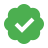# Supervisely/ Classification metricsCustom Updated An hour ago Free
Accuracy, precision, recall, F-measure

# Classification metrics

Studying classification metrics is better to start with the case of binary classification (i.e. when there are two classes). We will consider an example of a binary image classification, where the goal is to determine whether a dog is in the picture or not. If there is a dog in the pictures, then we will mark it with the tag "dog" and if not “no dog”.

To describe the main classification metrics it is worthwhile to introduce the following definitions:

True Positives (TP): True positives are the cases when the actual class of the data point was 1(True) and the predicted is also 1(True). (If there is a dog in the picture and we tag it as “dog”)True Negatives (TN): True negatives are the cases when the actual class of the data point was 0(False) and the predicted is also 0(False) (If there are no dogs in the picture and we tag it as “no dog”)False Positives (FP): False positives are the cases when the actual class of the data point was 0(False) and the predicted is 1(True). False is because the model has predicted incorrectly and positive because the class predicted was a positive one(1). (If there are no dogs in the picture and we tag it as “dog”False Negatives (FN): False negatives are the cases when the actual class of the data point was 1(True) and the predicted is 0(False). False is because the model has predicted incorrectly and negative because the class predicted was a negative one(0). (If there is a dog in the picture and we tag it as “no dog”)They all make up the Confusion matrix:The most common classification metric is Accuracy, it is determines as the ratio between the number of correct predictions and the total number of predictions made, or in terms of confusion matrix:Another popular metric is Precision:Precision talks about how precise/accurate your model is out of those predicted positive, how many of them are actual positive. (In our case: what fraction of the pictures we tagged as "dog" actually contains dogs.)

Often, a metric such as Recall is used with Precision:Recall is the fraction of relevant instances that have been retrieved over the total amount of relevant instances. (In our case: what fraction of the pictures containing dogs we tagged as “dog”)

If you want to seek a balance between Precision and Recall, you can use F1-Measure(or F1-Score), which is a function of these two quantities:### Multiclass case

All these metrics can also be defined in the multi-class setting. Here, the metrics can be "averaged" across all the classes in many possible ways. Some of them are:

• micro: Calculate metrics globally by counting the total number of times each class was correctly predicted and incorrectly predicted.

• macro: Calculate metrics for each "class" independently, and find their unweighted mean. This does not take label imbalance into account.

## Config example

``````{
"project_gt": "pr1_name",
"project_pred": "pr2_name",
"confidence_threshold": 0.5,
"tags_mapping": {
"pr1_cls_name_1": "pr2_cls_name_1",
"pr1_cls_name_2": "pr2_cls_name_2"
}
}
``````
1. `tags_mapping` - This section defines a mapping between tag names that denote the same class in the two respective projects. Only tags from this mapping will be considered for calculating metrics, all other tags will be ignored.

2. `confidence_threshold` - For tags with numeric values, use this value as a confidence threshold. Only the tags with values no less than the threshold will be used for metrics computation. For all other tag types, no thresholding is done.

3. `project_gt`(`project_pred`) - name of 1st(2nd) project involved in metrics evalutaion. Now Classification metrics plugin operate only with image tags:### Docker Image

`supervisely/metric-class-metrics:latest`

ID
75273
Version ID
84500
First released
5 months ago
Last updated
An hour ago

s